Online Writing Support
OWS HOME               PARTS OF SPEECH               SENTENCE STRUCTURE               PUNCTUATION             USAGE             SELF-TEACHING UNITS             EXERCISES

# Sentence patterns

Just about all sentences in the English language fall into ten patterns determined by the presence and functions of nouns, verbs, adjectives, and adverbs.

The patterns are most easily classified according to the type of verb used:

Verb of being patterns (1, 2, 3) use a form of the verb to be as the main verb in the sentence.

is      are      was      were      has been     have been      had been

Linking verb patterns (4, 5) use one of the linking verbs as the main verb in the sentence.  The linking verb is followed by a noun or adjective functioning as a subjective complement.

smell     taste     look     feel     seem     become      appear     grow

Action verb patterns (6, 7, 8, 9, 10) use one of the many action verbs as the main verb in the sentence.  The action verb may be either transitive (take a direct object) or intransitive (not take a direct object).

see    jump      embrace    write    imagine    buy    plummet    think  etc.

Terms used to identify various parts of each sentence pattern include the following:

• NP = noun phrase

This abbreviation refers to a headword noun and its modifiers ("noun phrase") functioning as a subject, direct object, indirect object, subjective complement, or objective complement.

• NP1, NP2, NP3, etc. = designations for different noun phrase functions

Numbers in sequential order are used with each NP to designate its difference from or similarity to other NPs before and after it.

• V-be = verb of being

• V-int = intransitive verb

• V-tr = transitive verb

#### THE TEN SENTENCE PATTERNS

1.  NP1 + V-be + ADV/TP

The verb of being is followed by an adverb indicating where or when.More information on subjects

The adverbial indicating where or when may be a prepositional phrase.2.  NP1 + V-be + ADJ

The verb of being is followed by an adjective that functions as the subjective complement.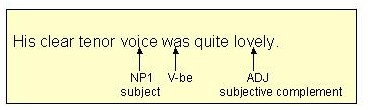More information on subjective complements

The adjectival functioning as the subjective complement may be a prepositional phrase.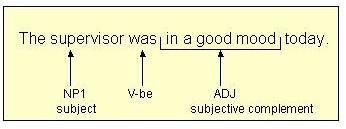3.  NP1 + V-be + NP1

The verb of being is followed by a noun that functions as the subjective complement.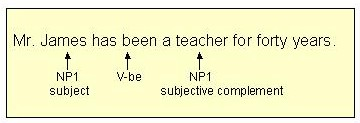Note:  The second NP receives the same numerical designation as the first NP because the second NP, the subjective complement, is the same as the subject (Mr. James = teacher).

4.  NP1 + LV + ADJ

The linking verb is followed by an adjective functioning as a subjective complement.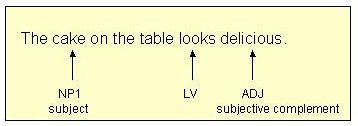The adjectival functioning as the subjective complement may be a prepositional phrase.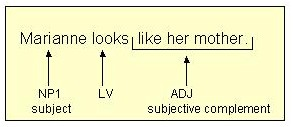5.  NP1 + LV + NP1

The linking verb is followed by a noun functioning as a subjective complement.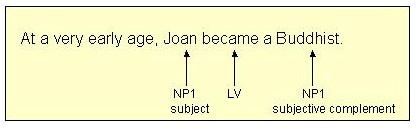Note:  The second NP receives the same numerical designation as the first NP because the second NP, the subjective complement, is the same as the subject (Joan = Buddhist).

6.  NP1 + V-int

The action verb takes no direct object.Even if the action verb is followed by a prepositional phrase, the verb is still intransitive as long as it does not take a direct object.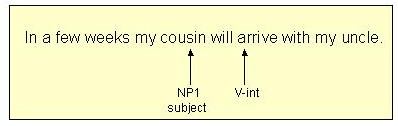7.  NP1 + V-tr + NP2

The action verb is followed by a direct object.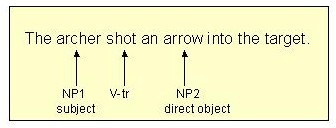More information on  direct objects

Note:  The second NP, the direct object, receives a different numerical designation (NP2) because it is not the same as the subject (NP1).

8.  NP1 + V-tr + NP2 + NP3

The action verb is followed by an indirect object and then a direct object.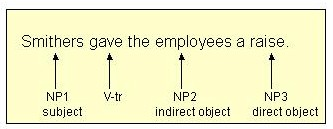More information on indirect objects

Note:  The indirect object and the direct object each receive a new numerical designation because each is different from the other and both are different from the subject.

9.  NP1 + V-tr + NP2 + ADJ

The action verb is followed by a direct object.  The direct object is followed by an adjective functioning as an objective complement.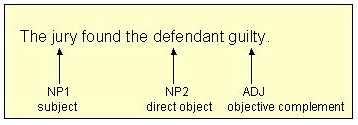More information on objective complements

Note:  The second NP, the direct object, receives a different numerical designation (NP2) because it is not the same as the subject (NP1).

10. NP1 + V-tr + NP2 + NP2

The action verb is followed by a direct object.  The direct object is followed by a noun functioning as an objective complement.Note:  The second NP, the direct object, receives a different numerical designation (NP2) because it is not the same as the subject (NP1).  The third NP, the objective complement, receives the same numerical designation as the direct object (NP2) because it is the same as the direct object (Jacobsen = friend).

For further information on these resources, contact
Margaret L. Benner
benner@towson.edu